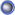Thread: My anti seismic systems View Single Post
#103seismicRegistered User Join Date: Feb 2010 Location: ISLAND OF IOS CYCLADES Posts: 107
Many researchers and engineers tell me that the explanations I give for my patent are insufficient and that they all need mathematical documentation. I answer them.

Mathematics is well known and can be done by those who have learned it. Simple assumptions of engineering are.

What is not known is what I am saying.

What am I saying. I come up with new methods of planning, explaining how I divert forces and where direct them. Basically I show them the design methods

And some simple mathematics.

The wall is a huge lever arm

To find the rotation of a wall, which is a lever arm, we must first find the force applied to it and multiply it by its distance from the joint of the base.

To find the force which is the force from inertia, multiply the mass of the structure by acceleration.

So, if we have the lateral force of inertia at the upper end of the wall, and the distance from the base joint, then multiplying these two numbers, we find the force of rotation of the wall.

The mathematical result of the force of rotation is divided by the distance the tendon has from the base joint, and we find the force taken by the tendon, which it carries from the top end of the wall into the ground.

Example

The drawing shows three walls of different dimensions in width.

On all walls, a lateral force of 40 tons applies. This force tends to rotate them around the joint of the base tread.

In the first left figure we see a tendon, without affinity, in the center.

The other two walls of the drawing have two tendons each near their sides.

Required. What should be the stability force (A) on the first wall, the stability force (B) on the second wall, and the stability force (C) on the third wall so that they do not overturn?
It must be ... The Torsion Torque <less than the Torque of Stability.

So

Wall stabilization torque (A)> 40X3.5 / 0.6 = 233.3 tonnes

Wall stabilization torque (B)> 40X3.5 / 1.5 = 93.3 tonnes

Wall stabilization torque (Γ)> 40X3.5 / 1.8 = 77.7 tonnes.
Here we observe that, as the distance between the tendon and the joint increases, the inversion torque decreases.
For this reason, we conclude that the invention is more efficient on adhesive walls than on columns.